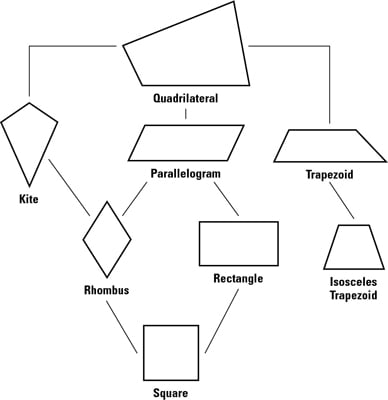##### Trigonometry Workbook For DummiesThe quadrilateral family tree in the figure below shows you the relationships among the various quadrilaterals. The following list of questions gives you a taste of some of these relationships. (You can test yourself by supplying the answers — always, sometimes, or never — before you check them.)• Is a rectangle a rhombus?

Sometimes (when it’s a square).

• Is a kite a parallelogram?

Sometimes (when it’s a rhombus).

• Is a rhombus a parallelogram?

Yes, always.

• Is a kite a rectangle?

Sometimes (when it’s a square).

• Is a trapezoid a kite?

No, never.

• Is a parallelogram a square?

Sometimes.

• Is an isosceles trapezoid a rectangle?

No, never.

• Is a square a kite?

Yes, always.

• Is a rectangle a square?

Sometimes.

Keep your family tree (in the above figure) handy when you’re doing always, sometimes, never problems because you can use the quadrilaterals’ positions on the tree to figure out the answer. Here’s how:

• If you go up from the first figure to the second, the answer is always.

• If you go down from the first figure to the second, then the answer is sometimes.

• If you can make the connection by going down and then up (like from a rectangle to a kite or vice versa), the answer’s sometimes.

• If the only way to get from one figure to the other is by going up and then down (like from a parallelogram to an isosceles trapezoid), the answer is never.